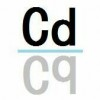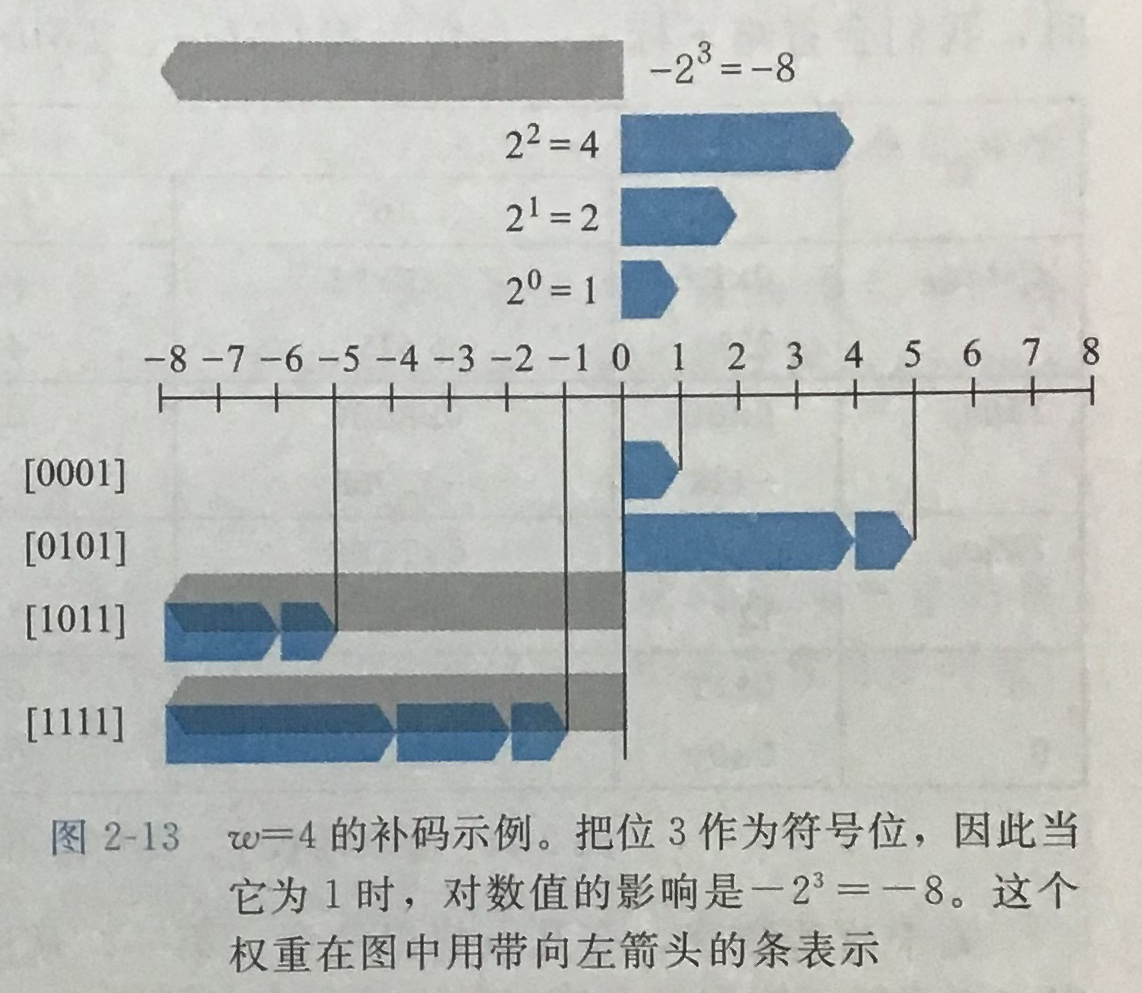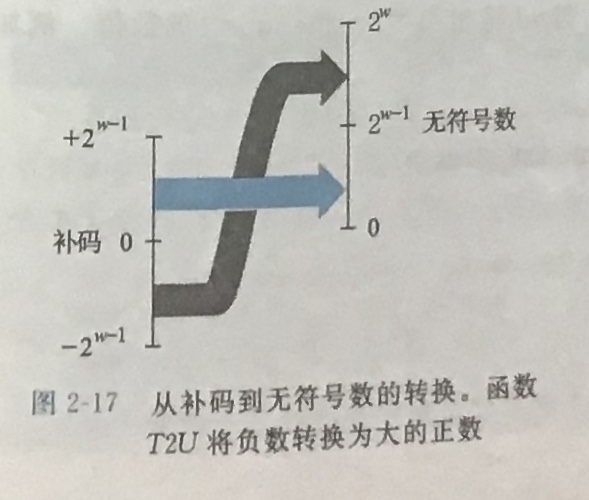2019-12-23 21:45:45$-A$是负数，所以其最高位应为$x_{w-1}=1$，不妨将$A$中的最高位变为1，其余位不变，观察其变化。

$B$是$A$除最高位取反的结果，而$B'$是$B$最高位取反的结果，那么$B'$就是$A$全部取反的结果，所谓取反再加一的计算方法可以由此推来。

$-2^{w-1}+2^{w-2}=-2^{w-2}$，这就等价于最高位为$w-2$位的二进制负数。

（在C语言中）

1. 为什么abs(-2147483648)=-2147483648？

2. 什么情况下i<=strlen(b)-1会出现错误？应该怎么更改以避免这个错误？（提示：strlen()函数的返回值为无符号整数）

3. 为什么在C语言的limits.h头文件中要把int类型变量的最小值INT_MIN定义为(-INT_MAX-1)，而不是直接定义为-2147483648？

• star
首页